Email   Home Page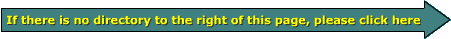Ohm's Law

There are 2 base formulae which will help you to understand the relationship between current , voltage, resistance and power. If you have any two of the parameters, you can calculate the other two parameters.

 OHM'S LAW BASE FORMULAS P=I*E E=I*R TO FIND VOLTAGE E=P/I E=I*R E=SQR(P*R) TO FIND CURRENT I=P/E I=E/R I=SQR(P/R) TO FIND POWER P=I*E P=E 2 /R P=I 2 *R TO FIND RESISTANCE R=E 2 /P R=E/I R=P/I 2

 P = Power in Watts E = Electromotive Force in Volts I = Electrical Current in Amps R = Electrical Resistance in Ohms SQR = Square Root Note: 'E' is generally used to represent voltage but sometimes you'll see 'V' used for voltage. Don't let it confuse you.

Short Refresher Course

Change of Resistance:
In the following diagram, you can see that the only difference between the diagrams on the left and the diagrams on the right is the resistance in each 'system'. The resistance in the faucet corresponds to the amount that the valve is open. In the wire, the resistance is the size of the opening* in the piece of wire. You can see that the voltage/pressure is the same for both the left and right examples. What you should note in this diagram is... All else being equal, if there is an increase in resistance, the current flow will be reduced. You can see that the current flow in the rightmost wire is half of the current flow in the leftmost wire. This is because the rightmost wire has half of the area for the electrons to pass through.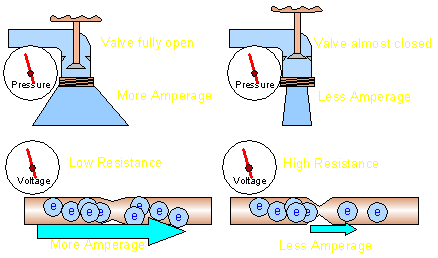*Please note that the size of the 'opening' is is analogous to resistance. There is no physical restriction in a real piece of wire.

With the formula:
I = E/R
You can see that the current flow is inversely proportional to the resistance in the circuit.

More Resistance = Less Current

And for those who are more graphically inclined...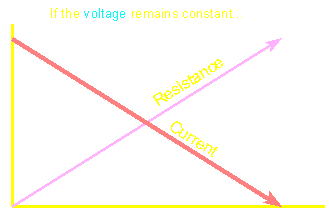Change of Voltage:
In the following diagram, you can see that the resistance in all of the systems is equal. This time we made a change in the voltage/pressure. You can see that the increased voltage causes an increase in current even though the resistance in both the left and right systems is equal.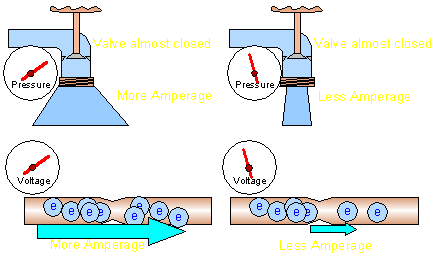With the formula:
I = E/R
You can see that the current flow is directly proportional to the voltage applied to the resistance.

More Voltage = More Current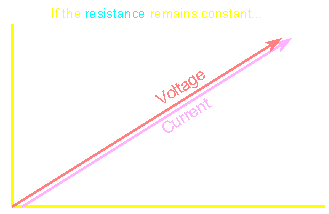Well, now that that's been explained to death, onto the math!

Mathematical Example: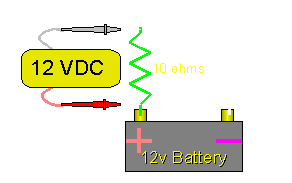In the following example, we know that we have 12 volts applied to a 10 ohm resistor. If you want to know how much power dissipation there is in the 10 ohm resistor, use the formula:

P = E2/R
P = 122/10
P = 144/10.
P = 14.4 watts

The power dissipation in the resistor is 14.4 watts.

If you wanted to know how much current was flowing through the resistor, you would use the formula:

I = E/R
I = 12/10
I = 1.2 amps

The current through the resistor is 1.2 amps.

If you want more examples, the resistor page has more fun than a barrel of monkeys.

If you want to try a few for yourself, the calculators below will allow you to check your math.

Find: Power dissipation and Current flow from Resistance and applied Voltage.

 Data Input: Resistor Value? = Ohms Applied Voltage? = Volts Data Output: Power Dissipation = Watts Current Flow = Amps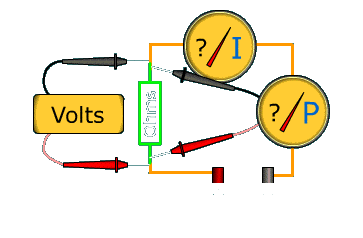Find: Circuit Resistance from Current Flow and applied Voltage.

 Data Input: Applied Voltage? Volts Current Flow? Amps Data Output: Resistor Value = OhmsFind: Applied Voltage and Current Flow from Power Dissipation and Circuit Resistance.

 Data Input: Power Dissipation? = Watts Resistor Value? = Ohms Data Output: Applied Voltage = Volts Current Flow = Amps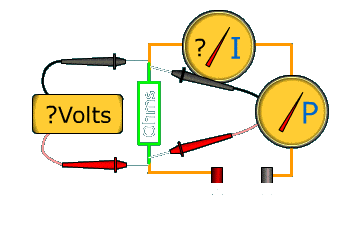----- Critically Important -----
Adobe has deemed that the Flash content on web pages is too risky to be used by the general internet user. For virtually all modern browsers, support for Flash was eliminated on 1-1-2021. This means that those browsers will not display any of the interactive Flash demos/calculators/graphics on this (or any other) site.
The simplest (not the best) fix, for now, is to download the Ruffle extension for your browser. It will render the Flash files where they were previously blocked. In some browsers, you will have to click on the big 'play' button to make the Flash applets/graphics visible.
An alternative to Ruffle for viewing Flash content is to use an alternative browser like the older, portable version of Chrome (chromium), an older version of Safari for Windows or one of several other browsers. More information on Flash capable browsers can be found HERE. It's not quite as simple as Ruffle but anyone even moderately familiar with the Windows Control Panel and installation of software can use Flash as it was intended.

Note:
In the following demo, you can adjust the voltage and the resistance of the circuit. Changing either one will change the current flow and power dissipation in the circuit. You should realize that an amplifier produces a voltage into a given load. The combination of the two will result in power dissipation (in the speaker's voice coil in the case of audio). Since an amplifier can produce a limited amount of voltage (limited by the internal power supply's design), the power output is limited when driving a given load (i.e. a 4 ohm load). To be able to produce more power, you can drive a lower impedance (resistance) load (within reason). This means that if you go from a 4 ohm load to a 2 ohm load, the power will double (assuming that the power supply is regulated). If we use the example of a 100 watt mono amplifier (100 watts into a 4 ohm load), we know it can produce no more than 20 volts across the speaker. If you set the voltage slider below to 20 volts, and the resistance slider to 4, you can see that the power is 100 watts. If you move the resistance slider to 2 ohms, you can see that the power is doubled (to 200 watts). Now, I know that I'm showing a battery as the voltage source (instead of an amplifier) but the concept is the same.

Historical Information

Georg Simon Ohm:
Georg Simon Ohm was a German physicist that lived from 1789 to 1854. He discovered the relationship between voltage, current and resistance in a conductor of a constant temperature (constant temperature is important because resistance changes with temperature and Ohm's law doesn't deal with the changing temperature/resistance). He found that, with a constant resistance, voltage and current were directly proportional (as we showed in a graph above). This relationship can be expressed as V=IR where V is the voltage applied to the resistance, I is the current flow through the resistance and R is the resistance in ohms.

James Watt:
James Watt was a Scottish inventor that lived from 1736 to 1819. The unit of measure for power, the watt, was named in his honor.

James Prescott Joule:
James Prescott Joule was an English physicist that lived from 1818 to 1889. He discovered the relationship between the power dissipated in a resistor and the current flow through the resistor. This relationship can be represented by the formula P=I²R where P is the power dissipation in watts, I is the current flow in amps R is the resistance in ohms. Ohm is generally given credit for the formulas which express the relationship between Power, Current, Resistance and Voltage but the credit probably should go to Joule.

The 'Joule' as a unit of measure:
A 'Joule' represents the amount of energy used when 1 watt is dissipated for 1 second (or 1 watt-second).

 You should remember: 1.If you have any 2 of the 4 electrical properties, you can find the other properties through Ohm's law.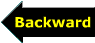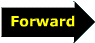```

```